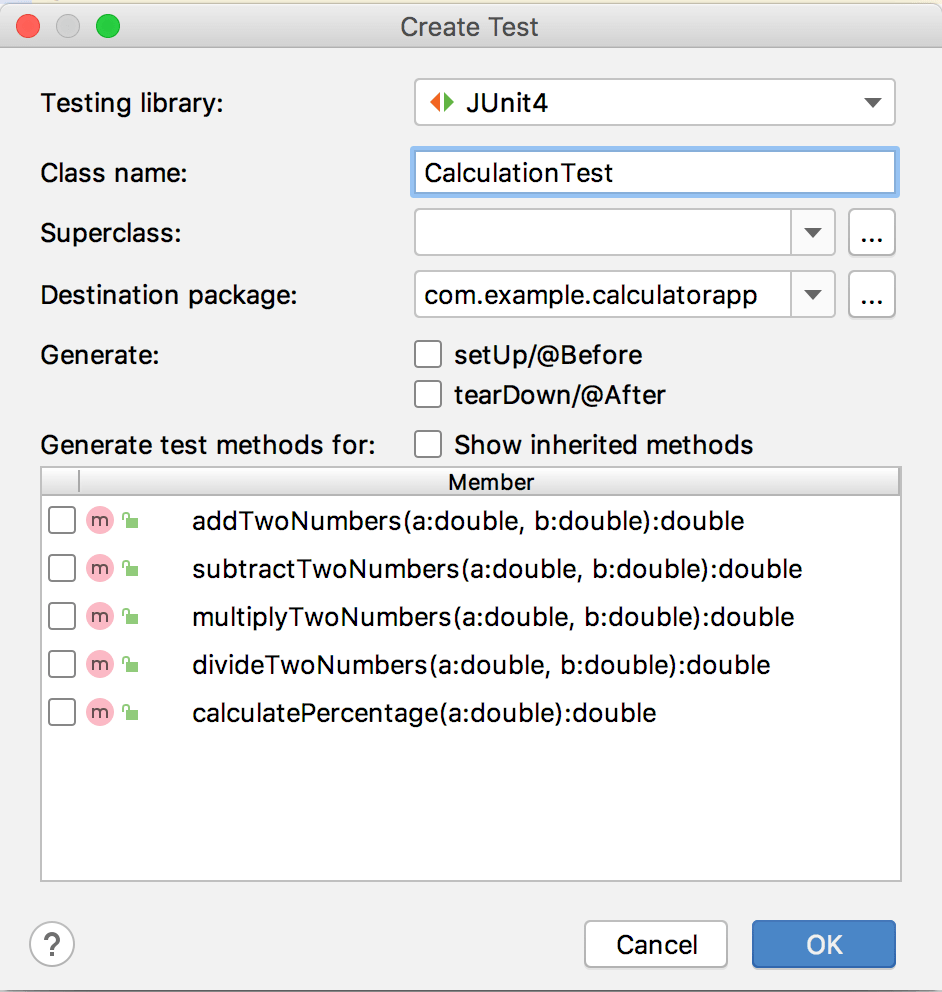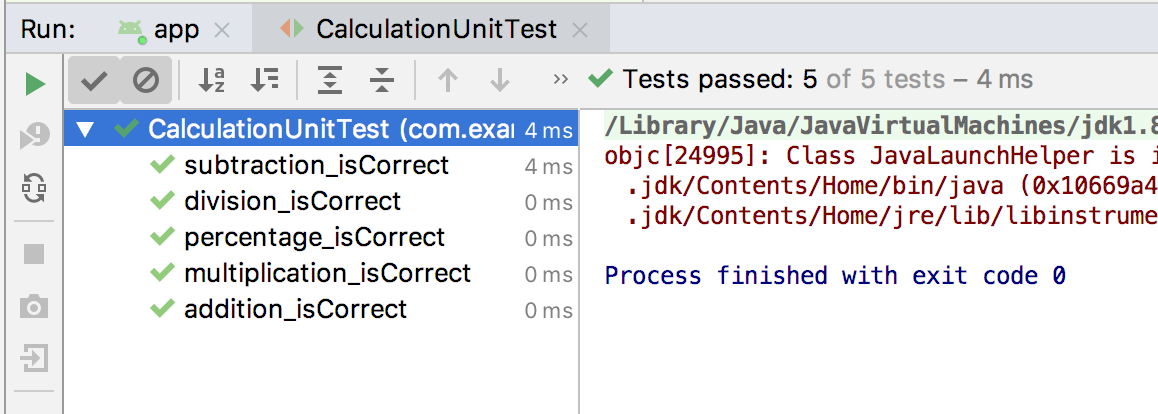# Android Junit and Espresso Testing using CalculatorApp example

CalculatorApp will perform operations like addition, subtraction, multiplication, division, percentage only on two numbers. In CalculatorApp we will write Unit and UI test cases on all the operations.

When you run the application CalculatorApp it will give you the output as shown below:

Get Complete GITHUB code from here.

### Testing

By running tests against your app consistently, you can verify your app’s correctness, functional behavior, and usability before you release it publicly.

A typical project in Android Studio contains two directories in which you place tests.

• The androidTest directory should contain the tests that run on real or virtual devices. Such tests include integration tests, end-to-end tests, and other tests where the JVM alone cannot validate your app’s functionality.
• The test directory should contain the tests that run on your local machine, such as unit tests.

### Testing Tools in android

There are many tools that can be used for testing android applications. Some are official like Junit, Espresso and some are third-party tools that can be used to test android applications. In this article, we will talk about these two tools to test android applications.

• Junit
• Espresso

#### JUnit

Junit is a “Unit Testing” framework for Java Applications.

Unit testing is a way of testing the smallest piece of code referred to as a unit (i.e method, class, component) that can be logically isolated in a system.

It is mainly focused on the functional correctness of standalone modules.

Unit testing consists of test cases that are used to check the business logic of your code.

#### Espresso

It is used to automate manual test cases for Android applications.

Espresso automatically synchronizes your test actions with the UI of your application. The framework also ensures that your activity is started before the tests run and also lets the test wait until all observed background activities have finished.

If you are new to Espresso then refer to my previous article Android Espresso Testing Basics .

The main components of Espresso include the following:

• Espresso – Entry point to interactions with views (via onView() and onData()).
• ViewMatchers –(Match a view) Allows you to find  view in the current view hierarchy.
• ViewActions – (Perform an action)Allows you to perform an action on a View.
• ViewAssertions – (Assert and verify the result)Check the state of the View to see if it reflects the expected state or behavior defined by the assertion.

Now let’s start creating our CalculatorApp example.

### Creating CalculatorApp

1 . In our CalculatorApp inside main>java we will create one java file Calculation.Java in which we will define all the operations (ie. addition , subtraction, multiplication , division, percentage) which we want to perform on two numbers.

Calculation.Java

```package com.example.calculatorapp;

public class Calculation {

public double addTwoNumbers(double a, double b) {
return a + b;
}

public double subtractTwoNumbers(double a, double b) {
return a - b;
}

public double multiplyTwoNumbers(double a, double b) {
return a * b;
}

public double divideTwoNumbers(double a, double b) {
return a / b;
}

public double calculatePercentage(double a) {
return a / 100;
}

}```

2 .To create Unit and UI tests cases for the operations defined in Calculation class we will create two classes:

• CalculationUnitTest under test folder for writing Unit tests.
• CalculationTest under androidTest folder for writing UI tests.

3 .Now right-click on Calculation class and select Generate..>Tests..4 .A new Create Test window will appear as shown below:5 .In above window provide Test class name and check all the methods which you want to use in your class and then click OK.

6 .Now choose the destination directory ie. androidTest or test in which you will add the above-defined class and then click OK.After creating the TestClass you are ready to start writing your test cases.

7 .CalculationUnitTest consist of unit test cases for each operation defined with @Test notation.

CalculationUnitTest.Java

```package com.example.calculatorapp;

import org.junit.Test;

import static org.junit.Assert.*;

public class CalculationUnitTest {

@Test
}

@Test
public void subtraction_isCorrect() {
assertEquals(5, new Calculation().subtractTwoNumbers(8,3),0);
}

@Test
public void multiplication_isCorrect() {
assertEquals(24, new Calculation().multiplyTwoNumbers(8,3),0);
}

@Test
public void division_isCorrect() {
assertEquals(3, new Calculation().divideTwoNumbers(9,3),0);
}

@Test
public void percentage_isCorrect() {
assertEquals(0.03, new Calculation().calculatePercentage(3),0);
}

}

```

Each test consist of assertEquals() which takes three inputs:

• expected value.
• actual value to check against expected value.
• delta ie. the maximum delta between expected and
actual value for which both numbers are still
considered equal.

8 .To run all unit test cases right-click on CalculationUnitTest class and then select Run ‘CalculationUnitTest’.9 .CalculationTest consist of UI test cases for all the operations.

• @RunWith : specifies the behavior for our TestClass.To create an instrumented JUnit 4 test class, add the @RunWith(AndroidJUnit4.class) annotation at the beginning of your test class definition.
• @Rule : Provides functional testing of a single activity. UI can access the activity using the rule and thereby can access the resources etc.
• @Test : The @Test annotation tells JUnit that the public void method to which it is attached can be run as a test case.

CalculationTest.Java

```package com.example.calculatorapp;

import org.junit.Rule;
import org.junit.Test;
import org.junit.runner.RunWith;

import androidx.test.ext.junit.runners.AndroidJUnit4;
import androidx.test.rule.ActivityTestRule;

import static androidx.test.espresso.Espresso.onView;
import static androidx.test.espresso.action.ViewActions.click;
import static androidx.test.espresso.assertion.ViewAssertions.matches;
import static androidx.test.espresso.matcher.ViewMatchers.withId;
import static androidx.test.espresso.matcher.ViewMatchers.withText;

@RunWith(AndroidJUnit4.class)
public class CalculationTest {

@Rule
public ActivityTestRule<MainActivity> activityScenarioRule
= new ActivityTestRule<>(MainActivity.class);

@Test
onView(withId(R.id.btn_two)).perform(click());
onView(withId(R.id.btn_plus)).perform(click());
onView(withId(R.id.btn_three)).perform(click());
onView(withId(R.id.btn_equalsto)).perform(click());
onView(withId(R.id.edt_calculation)).check(matches(withText("5")));

}

@Test
public void subtractTwoNumbers() {
onView(withId(R.id.btn_eight)).perform(click());
onView(withId(R.id.btn_minus)).perform(click());
onView(withId(R.id.btn_three)).perform(click());
onView(withId(R.id.btn_equalsto)).perform(click());
onView(withId(R.id.edt_calculation)).check(matches(withText("5.0")));

}

@Test
public void multiplyTwoNumbers() {
onView(withId(R.id.btn_eight)).perform(click());
onView(withId(R.id.btn_multiply)).perform(click());
onView(withId(R.id.btn_three)).perform(click());
onView(withId(R.id.btn_equalsto)).perform(click());
onView(withId(R.id.edt_calculation)).check(matches(withText("24.0")));
}

@Test
public void divideTwoNumbers() {
onView(withId(R.id.btn_nine)).perform(click());
onView(withId(R.id.btn_divide)).perform(click());
onView(withId(R.id.btn_three)).perform(click());
onView(withId(R.id.btn_equalsto)).perform(click());
onView(withId(R.id.edt_calculation)).check(matches(withText("3.0")));
}

@Test
public void calculatePercentage() {
onView(withId(R.id.btn_three)).perform(click());
onView(withId(R.id.btn_percentage)).perform(click());
onView(withId(R.id.edt_calculation)).check(matches(withText("0.03")));
}
}```
• onView() is used to select a view for testing.
• withId() is used to locate the UI element.
• perform(click()) is used to perform click operation.
• check(matches(withText())) is used to check whether the element is displayed with particular text or not.

10 .To run all UI test cases right-click on CalculationTest class and then select Run ‘CalculationTest’.### 1 thought on “Android Junit and Espresso Testing using CalculatorApp example”

1.Thanks for sharing such knowledgeable Content on Unit testing, to know how to enhance the performance of ERP’s like Oracle cloud through testing follow this link Oracle Cloud testing tools